# The Splitwise Problem

Oct 23, 2022

## Background

Splitwise is an app for keeping track of shared expenses on group trips. You enter all the transactions from the trip, and then the app will tell each person how much they owe each other person in the group.

In addition to being useful, Splitwise also inspires an interesting CS problem, which is: given a list of transactions, how do you efficiently calculate the minimum number of payments that are necessary to settle everyone's debts?

For example, let's say Alice, Bob, and Carol take a day trip. Alice covers breakfast which is $30 total, Bob covers lunch which is$60, and Carol covers dinner which is $90. This means that Bob and Carol each owe Alice$10 for breakfast, Alice and Carol each owe Bob $20 for lunch, and Alice and Bob each owe Carol$30 for dinner. However, the optimal solution is not to have each person pay everyone else, for a total of $$6$$ payments. Instead, Alice just needs to pay Carol $30, for a total of $$1$$ payment, to settle everyone's debts. To see why, observe that after Alice pays Carol$30, everyone will be out an equal amount of $60. This example shows why this problem is tricky - if $$A$$ owes $$B$$ and $$B$$ owes $$C$$, it can be optimal to have $$A$$ pay $$C$$ directly. I spent some time thinking about this problem. One observation is that the specific transactions don't actually matter, all that matters is each person's net amount, which can be positive or negative. If they are net positive, that means they owe money, and if they are net negative, that means they are owed money. By this convention, in our example above Alice is net$30, Bob is net $0, and Carol is net -$30, which makes it easy to see the optimal solution.

The answer may not always be so obvious though. If we had a group of six people with net values of $130,$150, $20, -$15, -$125, and -$160, it's not immediately clear what the minimum number of necessary payments is.

## Formalization of the "Splitwise Problem"

Based on our observation, we can formalize the "Splitwise problem" as follows:

Given a list of $$N$$ integers $$x_1 \cdots x_N$$ such that $$\sum x = 0$$, define an exchange operation as subtracting an integer from the value at one index and adding it to the value at another index. What is the minimum number of exchange operations that are needed to make all the values zero?

In this formalization, $$N$$ represents the number of people in the group, $$x$$ represents their net values, and exchanges represent payments.

There's also a decision variant of this problem, where instead of finding the minimum number of exchanges $$M$$, we want to determine whether a given number of exchanges $$E$$ is achievable. The decision variant is at most as hard as the original variant, because if we can calculate $$M$$, then we can easily determine whether $$E$$ is achievable just by comparing it with $$M$$. In the solution below, we will be working with the decision variant.

## Solution

It turns out, the decision variant of this problem is NP-complete! This means that there is no known polynomial time solution to the problem, and if a polynomial time solution were discovered, that would prove that $$P=NP$$. Whether or not $$P=NP$$ is one of the biggest unsolved problems in computer science, so trying to find a polynomial time solution to the Splitwise problem in this blog post probably won't be very fruitful. Instead, we'll just prove that the problem is NP-complete.

To start, we need to show that the problem is in NP, which means that if the answer to an instance of the Splitwise decision problem is "yes", then there is a proof that is verifiable in polynomial time. Indeed this is the case, because as proof we can provide the list of $$E$$ exchanges. To verify the proof, we just need to apply each exchange and check that all values are $$0$$ afterwards, which can be done in linear time with respect to $$E$$ and $$N$$.

The next part is trickier, which is to show that the problem is NP-hard. To do this, we need to "reduce" a known NP-hard problem to the Splitwise problem. For our proof, we will use the Subset sum problem (SSP), which is known to be NP-hard. Given an instance of SSP, we will provide a polynomial time algorithm to convert that instance into an instance of the Splitwise problem. The existence of this conversion implies that if the Splitwise problem is solvable in polynomial time, then SSP is also solvable in polynomial time, because we can just convert any instance of SSP into the Splitwise problem and solve that. Because there is no known polynomial time solution to SSP, it logically follows that there is no known polynomial time solution to the Splitwise problem either.

In the Subset sum problem, you are given a list of positive integers and a target, and the question is to decide whether any subset of the integers sums to precisely the target. Consider an instance of SSP with $$S$$ positive integers $$p_1 \cdots p_S$$ and target $$T$$. We will convert this to the following instance of the Splitwise problem: $N = S+2$ $x_i = p_i \forall 1 \le i \le S$ $x_{S+1} = -T$ $x_{S+2} = T - \sum p$ $E = S$ We will refer to the indices $$1 \le i \le S$$ as borrowers because they owe money and the indices $$S+1$$ and $$S+2$$ as lenders because they are owed money. This is a straightforward mapping so it can be done in polynomial time. Next we will prove that the answer to the instance of SSP is "yes" if and only if the answer to the corresponding Splitwise problem is "yes".

First let's prove the forward direction of the "if and only if": if the answer to the SSP instance is "yes", then the answer to the corresponding Splitwise instance is "yes". Let $$A$$ be the set of indices that obtain the target sum, i.e. $$\sum_{a \in A} p_a = T$$. Then, in the corresponding Splitwise instance, that means we can use $$|A|$$ exchanges to move all values at indices $$a \in A$$ to the value at index $$S + 1$$, and $$S - |A|$$ exchanges to move all values at indices $$a' \notin A$$ to the value at index $$S + 2$$, for a total of $$S = E$$ exchanges. This proves the forward direction.

Next let's prove the backwards direction: if the answer to the Splitwise instance is "yes", then the answer to the corresponding SSP instance is "yes". Because there are $$S$$ positive values in $$x$$ and only $$S$$ exchanges, that means each positive value must be part of exactly one exchange, which turns that positive value to zero. If there is an exchange from borrower $$i$$ to borrower $$j$$ of value $$x_i$$, and then an exchange from borrower $$j$$ to a lender (e.g. $$S+1$$) of value $$x_i+x_j$$, that is equivalent in number of exchanges to if $$i$$ and $$j$$ each paid that lender directly. Therefore, we can assume that every exchange is from a borrower to a lender with the entire value that the borrower originally owed. Then, we can just take the set of indicies $$a \in A$$ such that there is an exchange from $$a$$ to $$S+1$$. Because $$x_{S+1} = T$$, this set of indices gives us a subset that achieves the target sum in the corresponding SSP problem, which proves the backwards direction.

We have shown that the Splitwise problem is both NP and NP-hard, which means it is NP-complete.

## How does Splitwise work?

We've proven that the Splitwise problem is NP-hard, so Splitwise doesn't have an efficient polynomial time solution to minimize the number of payments (unless they've secretly proven $$P = NP$$). What do they do then? There's a couple possibilities - either they use an inefficient exponential-time algorithm, or they use a polynomial-time approximation algorithm. Let's figure out which is the case! First thing to note is that Splitwise by default doesn't actually try to minimize the number of payments, but offers the option to do so via a "simplify group debits" toggle: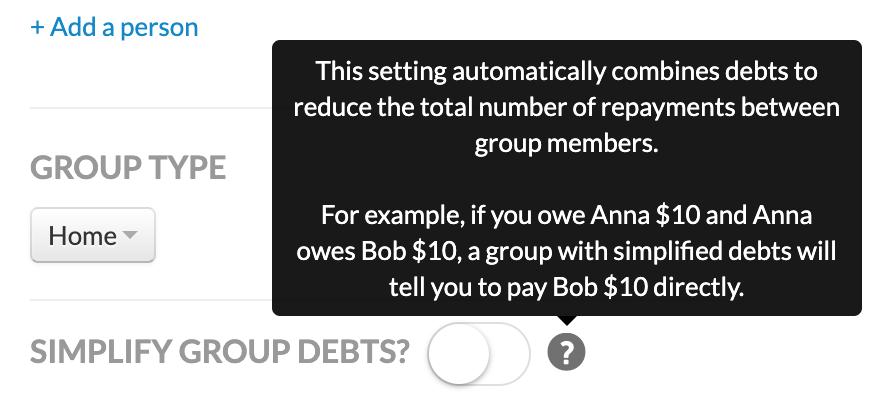Let's see how Splitwise handles our original example with Alice, Bob, and Carol. I'll replace Alice with myself. Here's the setup: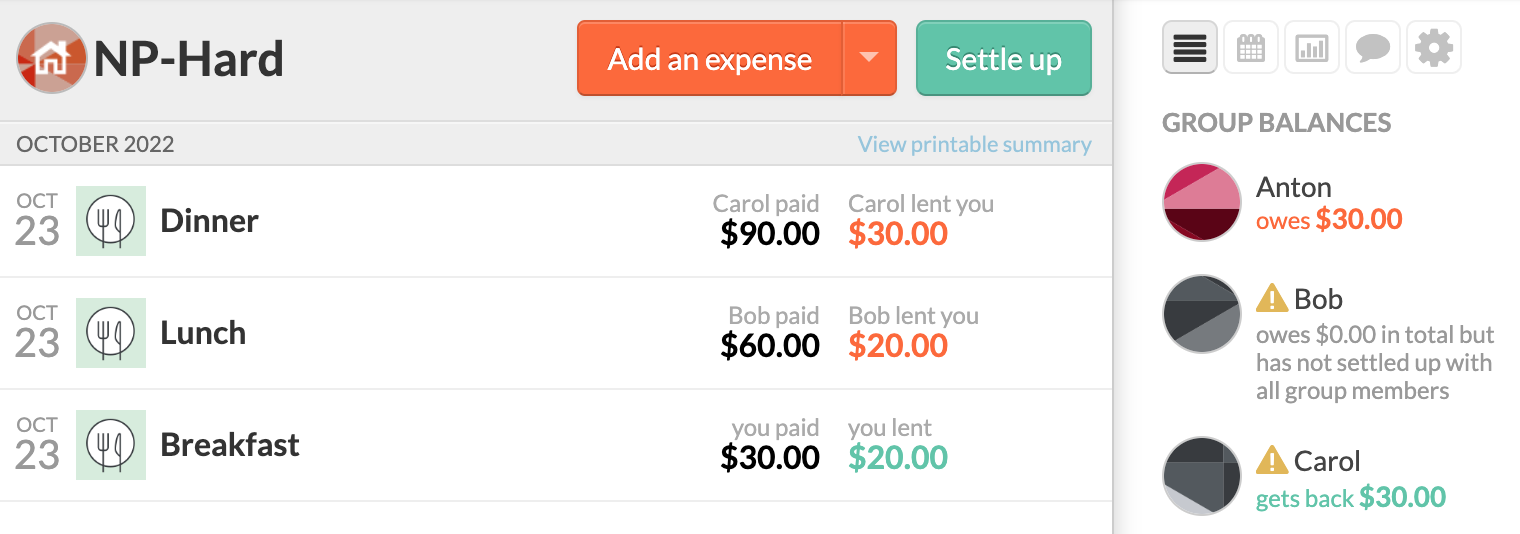With "simplify debts" disabled, Splitwise proposes that I pay Bob $10, I pay Carol$20, and Bob pays Carol $10, for a total of $$3$$ payments. So it doesn't return the optimal solution of $$1$$ payment, but it is better than the most naive solution of $$6$$ payments: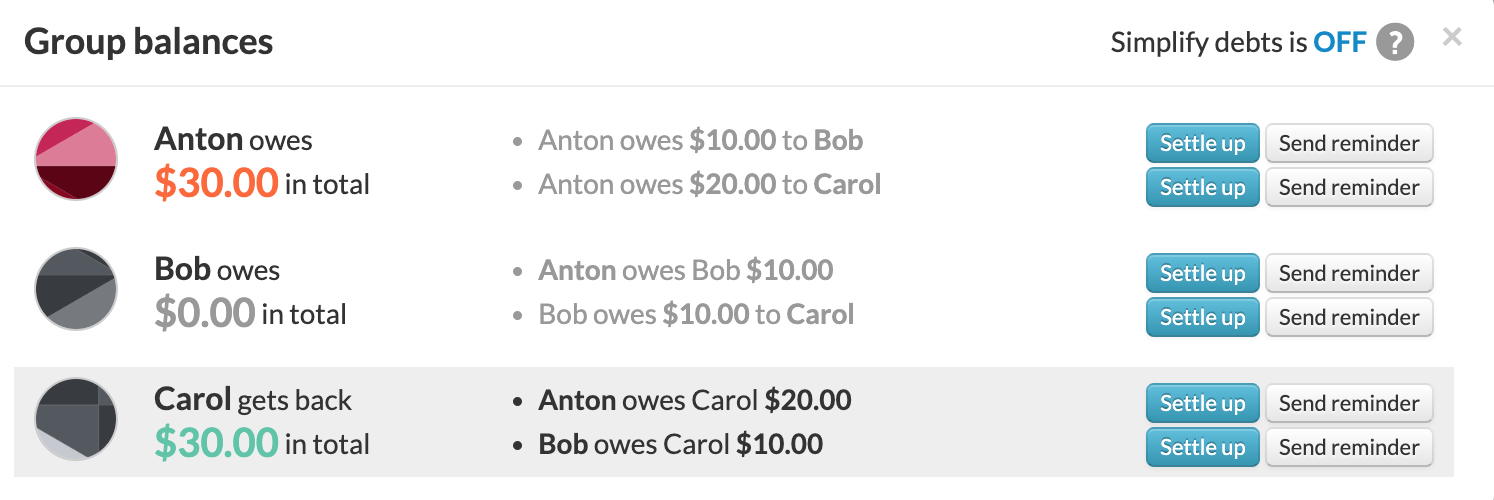With "simplify debts" enabled, Splitwise does propose the optimal solution of $$1$$ payment: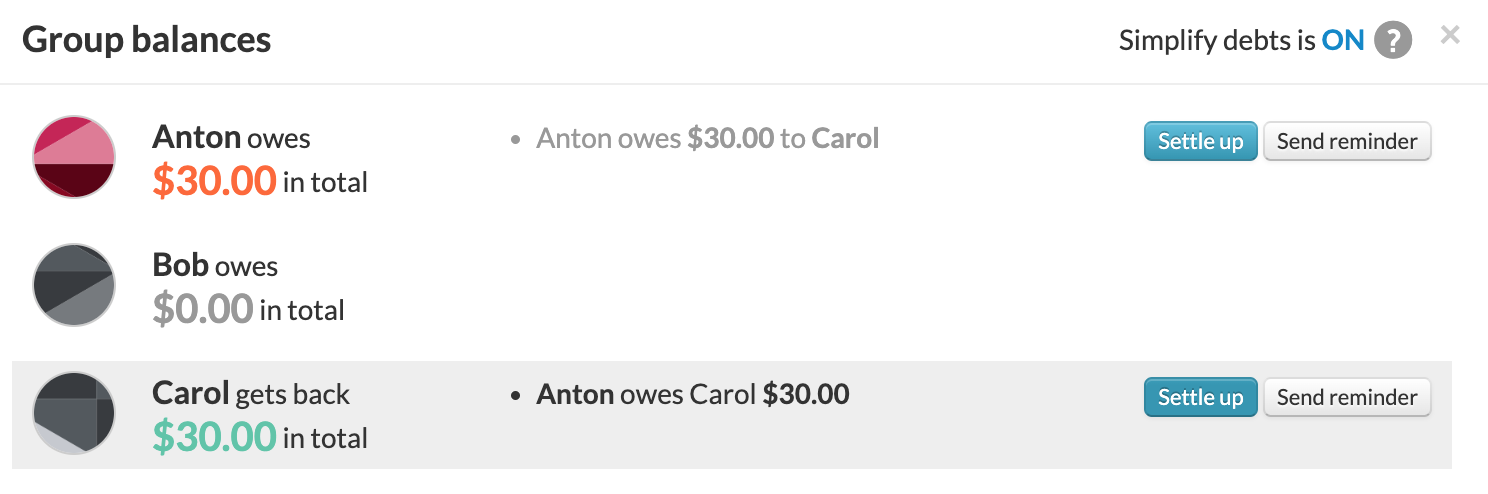Great! Now, let's try with a larger example that would be too expensive to compute exactly. I created a group with 42 members, labeled $$P_1 \cdots P_{40}$$, $$N_1$$, and $$N_2$$. The 40 labeled $$P$$ have a positive balance, and the 2 labeled $$N$$ have a negative balance. Specifically, each person $$P_i$$ owes $$N_{2 - (i \% 2)}$$ an amount equal to the $$i$$th prime number, e.g. $$P_1$$ owes $$N_1$$$2, $$P_2$$ owes $$N_2$$ $3, $$P_3$$ owes $$N_1$$$5, etc. In this example, the optimal number of payments is $$40$$, where each $$P_i$$ pays $$N_{2 - (i \% 2)}$$. However, Splitwise actually suggests $$41$$ payments by having $$P_{30}$$ split their payment between $$N_1$$ and $$N_2$$:Furthermore, Splitwise has $$P_1$$ through $$P_{30}$$ pay $$N_1$$, and $$P_{30}$$ through $$P_{40}$$ pay $$N_2$$: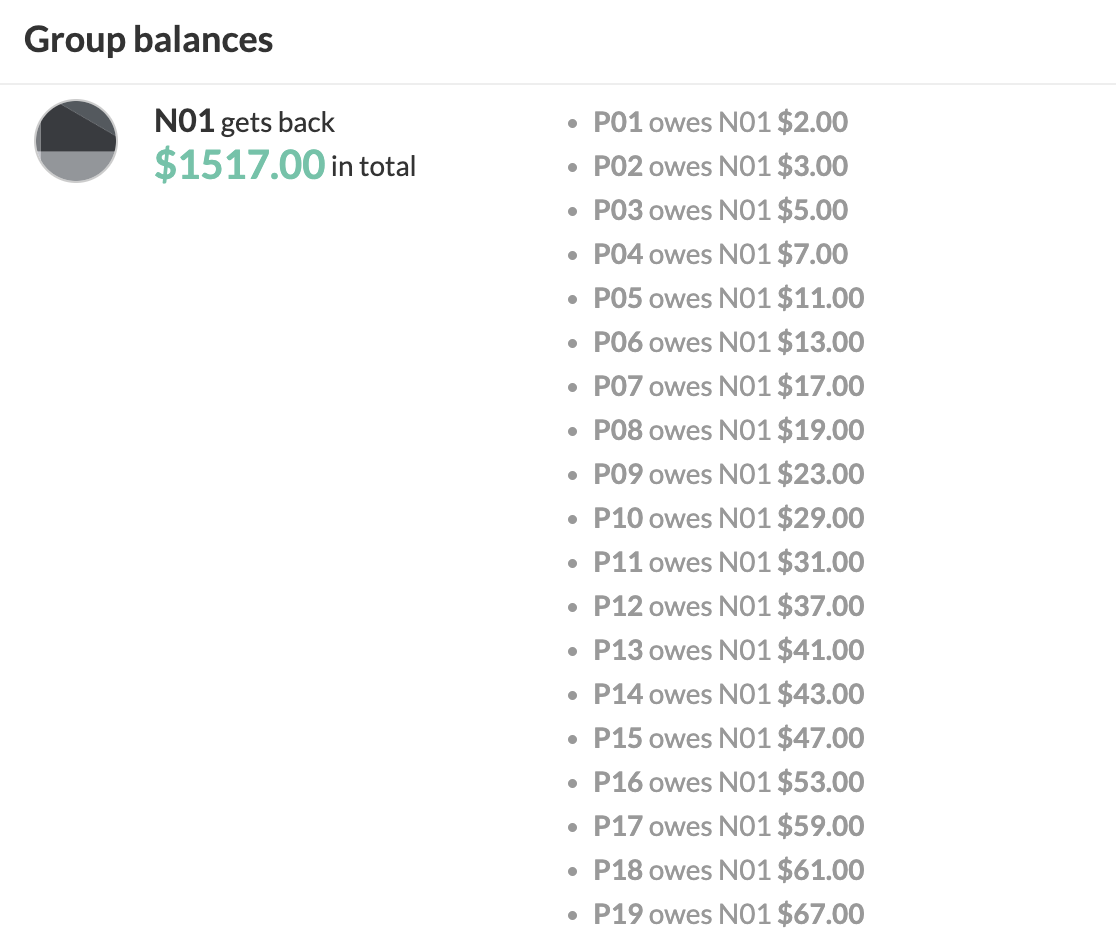Therefore, it appears that Splitwise uses a greedy algorithm that pays one lender at a time. It runs quickly but does not always produce the optimal answer.

The funny thing is that if you disable "simplfy debts" for this example then Splitwise will actually output the correct answer of $$40$$ payments :) This is because we only inputted 40 transactions, so Splitwise can just reverse each transaction. It's as if we gave Splitwise the "proof" that $$E = 40$$ is satisfiable, so it could verify it in polynomial time.